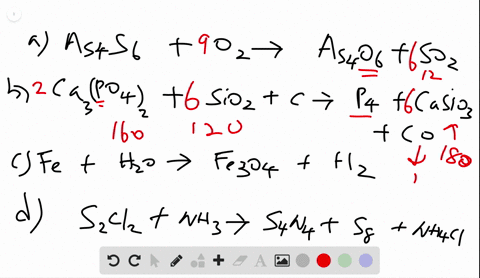Black Friday is Here! Start Your Numerade Subscription for 50% Off!Join Today### Write balanced equations for each of the followin…

08:59University of Toronto
Problem 58

# Write balanced equations for each of the following by inserting the correct coefficients in the blanks:(a) $_{-}\mathrm{SO}_{2}(g)+_{-} \mathrm{O}_{2}(g) \longrightarrow_{-}\mathrm{SO}_{3}(g)$(b) $_{-}\mathrm{Sc}_{2} \mathrm{O}_{3}(s)+_{-}\mathrm{H}_{2} \mathrm{O}(l) \longrightarrow_{-}\mathrm{Sc}(\mathrm{OH})_{3}(s)$(c) $_{-}\mathrm{H}_{3} \mathrm{PO}_{4}(a q)+_{-}\mathrm{NaOH}(a q) \longrightarrow{_{-}\mathrm{Na}_{2} \mathrm{HPO}_{4}(a q)+}_{-}\mathrm{H}_{2} \mathrm{O}(l)$(d) $_{-} \mathrm{C}_{6} \mathrm{H}_{10} \mathrm{O}_{5}(s)+_{-} \mathrm{O}_{2}(g) \longrightarrow_{-} \mathrm{CO}_{2}(g)+_{-} \mathrm{H}_{2} \mathrm{O}(g)$

## Discussion

You must be signed in to discuss.